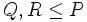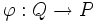# Fusion system

## Definition

The term fusion system is used for some additional structure (a category structure) on a finite p-group satisfying some conditions. There are two conflicting definitions of fusion system in use.

### Definition followed by Broto-Levi-Oliver

Broto-Levi-Oliver, Kessar, and others use the following convention: they define a fusion system as a category on a finite p-group that contains the inner fusion system. However, they do not include the two other axioms -- the Sylow condition and the extension axiom -- that form part of the saturated fusion system definition.

The two definitions are presented and contrasted below for$P$ a group of prime power order, say a finite$p$-group, for a prime$p$, and$\mathcal{F}$ a category on$P$:

Condition name Qualitative description of condition Condition details Part of the Linckelman definition of fusion system (same as the Broto-Levi-Oliver definition of saturated fusion system)? Part of the Broto-Levi-Oliver definition of fusion system?
contains inner fusion system This condition makes sure that the obvious homomorphisms are included For any subgroups$Q, R \le P$, all injective homomorphisms from$Q$ to$R$ that arise as restrictions of inner automorphisms of$P$, are present in$\mathcal{F}$. In other words, the inner fusion system on$P$ is a subcategory of$\mathcal{F}$. Yes Yes
Sylow axiom This condition is a purely global condition, i.e., it gives information only about automorphisms at the global level The inner automorphisms of$P$ form a Sylow subgroup of the group of automorphisms of$P$ in$\mathcal{F}$. Yes No
Extension axiom This condition links up the local and the global Call a subgroup$R$ of$P$ fully normalized by$\mathcal{F}$ if$|N_P(Q)| \le |N_P(R)|$ for any$Q \cong R$ where$\cong$ is isomorphism in the category$\mathcal{F}$.
Also define, for any morphism$\varphi:Q \to P$ in$\mathcal{F}$:$N_\varphi = \{ y \in N_P(Q) \mid \exists z \in N_P(\varphi(Q)), \varphi(yuy^{-1}) = z\varphi(u)z^{-1} \ \forall u \in Q \}$
Then the statement of the extension axiom is:
Every morphism$\varphi:Q \to P$ such that$\varphi(Q)$ is fully$\mathcal{F}$-normalized, extends to a morphism$\psi:N_\varphi \to P$.
Yes No

### Definition followed by Linckelman

Fusion systems as defined by Linckelman are what we here call saturated fusion systems. These are fusion systems that also satisfy the Sylow axiom and the extension axiom.

There are multiple conventions on whether the term fusion system should refer to saturated fusion system or to a weaker notion. For our purposes, we will mostly be interested in saturated fusion systems, because these mimic/generalize situations where the$p$-group is a$p$-Sylow subgroup of some finite group. Unless otherwise specified, we are referring to saturated fusion systems when we talk about fusion systems.

## Fusion systems induced by groups

Given any finite group$G$ and any$p$-subgroup$P$ of$G$, we can define the fusion system induced by$G$ on$P$. A case of special interest is where$P$ is a$p$-Sylow subgroup of$G$, and in that case we talk of the fusion system induced by a finite group on its p-Sylow subgroup. The following are true for fusion systems induced by finite groups on$p$-subgroups:

Condition on group or subgroup embedding Condition on fusion system Does the condition on the group/subgroup imply the condition on the fusion system induced? Given a fusion system with the condition, can we always find a group with the condition that induces that fusion system?
any any Yes May be, it's true for saturated fusion systems
the subgroup is a Sylow subgroup the fusion system is a saturated fusion system Yes No (saturated fusion systems for which this isn't true are termed exotic fusion systems)
the whole group is a simple group and the subgroup is Sylow the fusion system is a simple fusion system Yes No (?) Don't really know